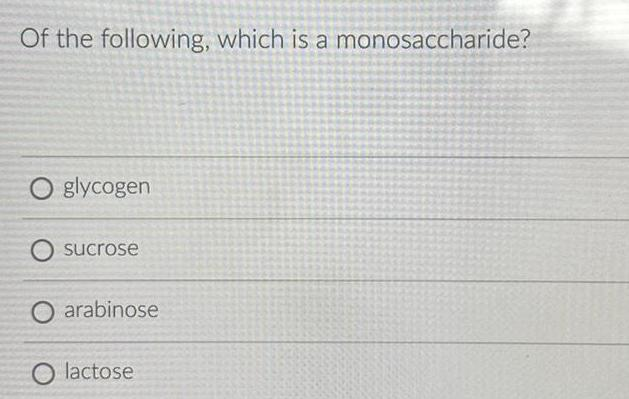Inorganic Chemistry
Hydrogen
Of the following, which is a monosaccharide? glycogen sucrose arabinose lactose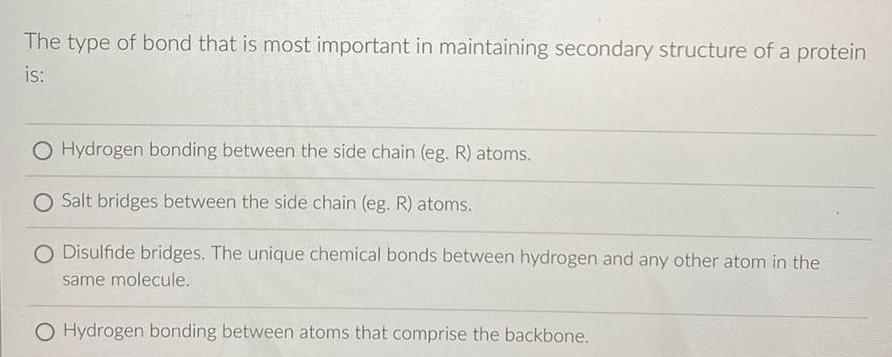Inorganic Chemistry
Hydrogen
The type of bond that is most important in maintaining secondary structure of a protein is: Hydrogen bonding between the side chain (eg. R) atoms. Salt bridges between the side chain (eg. R) atoms. Disulfide bridges. The unique chemical bonds between hydrogen and any other atom in the same molecule. Hydrogen bonding between atoms that comprise the backbone.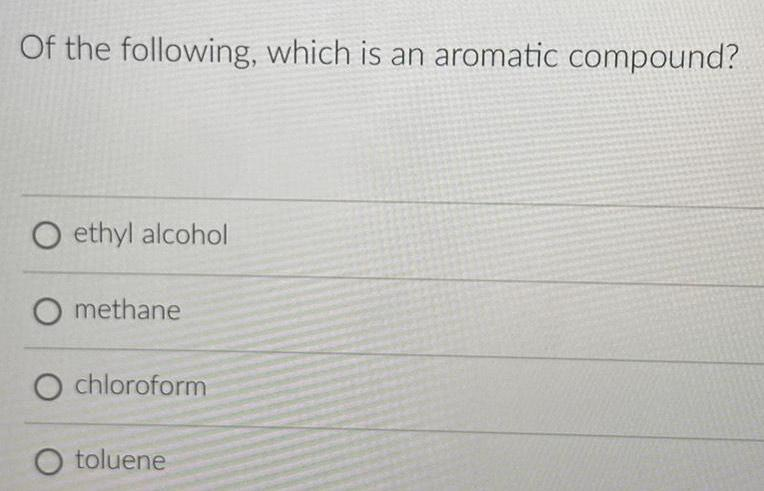Inorganic Chemistry
Hydrogen
Of the following, which is an aromatic compound? ethyl alcohol methane chloroform toluene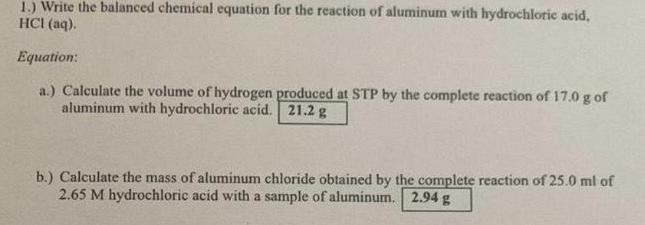Inorganic Chemistry
Hydrogen
Write the balanced chemical equation for the reaction of aluminum with hydrochloric acid, HCI (aq). Equation: a.) Calculate the volume of hydrogen produced at STP by the complete reaction of 17.0 g of aluminum with hydrochloric acid. b.) Calculate the mass of aluminum chloride obtained by the complete reaction of 25.0 ml of 2.65 M hydrochloric acid with a sample of aluminum.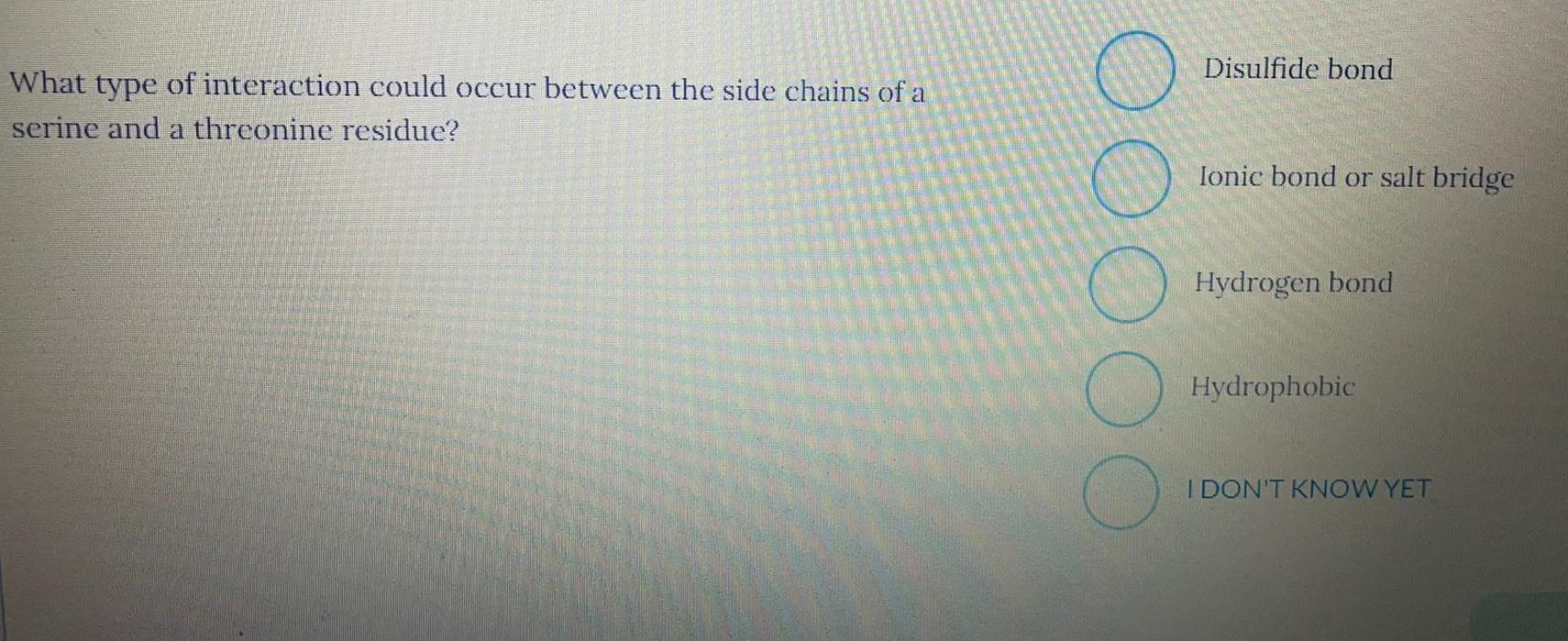Inorganic Chemistry
Hydrogen
What type of interaction could occur between the side chains of a serine and a threonine residue? Disulfide bond Ionic bond or salt bridge Hydrogen bond Hydrophobic I DON'T KNOW YET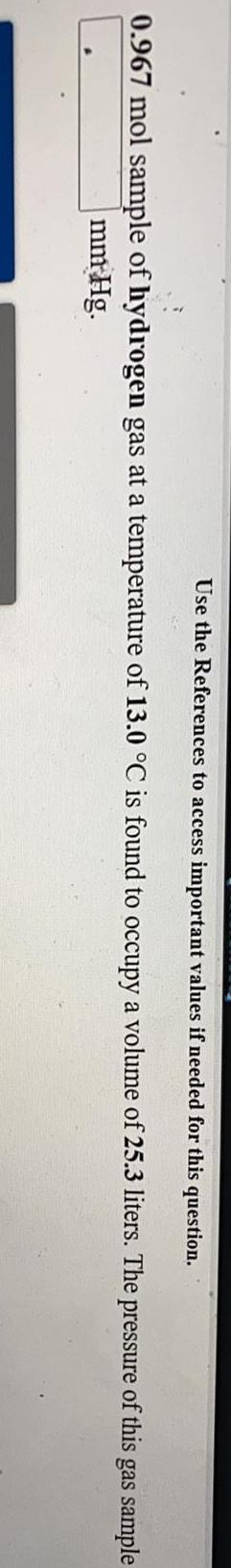Inorganic Chemistry
Hydrogen
0.967 mol sample of hydrogen gas at a temperature of 13.0 °C is found to occupy a volume of 25.3 liters. The pressure of this gas sample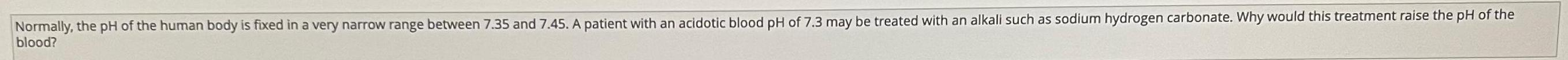Inorganic Chemistry
Hydrogen
Normally, the pH of the human body is fixed in a very narrow range between 7.35 and 7.45. A patient with an acidotic blood pH of 7.3 may be treated with an alkali such as sodium hydrogen carbonate. Why would this treatment raise the pH of the blood?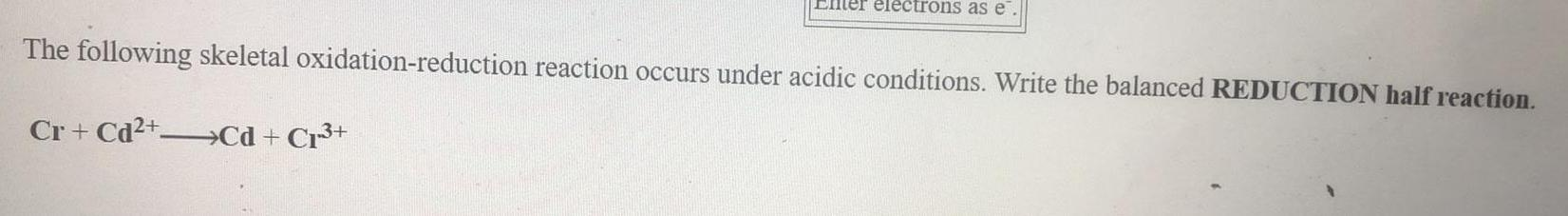Inorganic Chemistry
Hydrogen
The following skeletal oxidation-reduction reaction occurs under acidic conditions. Write the balanced REDUCTION half reaction. Cr+Cd²+ ---> Cd + Cr³+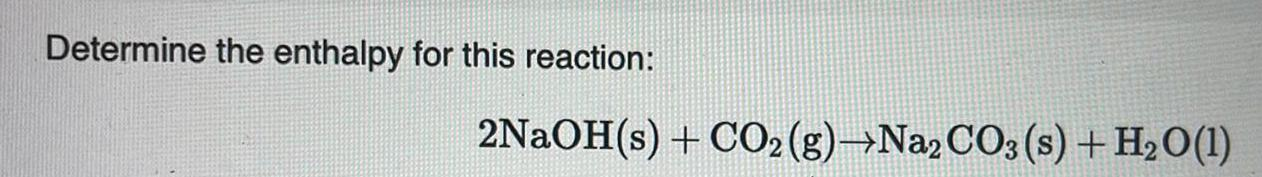Inorganic Chemistry
Hydrogen
Determine the enthalpy for this reaction: 2NaOH(s) + CO₂ (g)→Na2CO3 (s) + H₂O(l)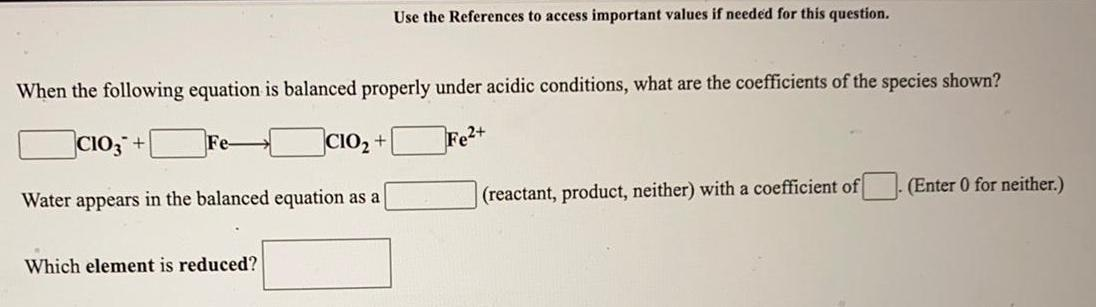Inorganic Chemistry
Hydrogen
When the following equation is balanced properly under acidic conditions, what are the coefficients of the species shown? CIO3 Fe= CIO₂ + Fe2+ Water appears in the balanced equation as a (reactant, product, neither) with a coefficient of. (Enter 0 for neither.)which element is reduced?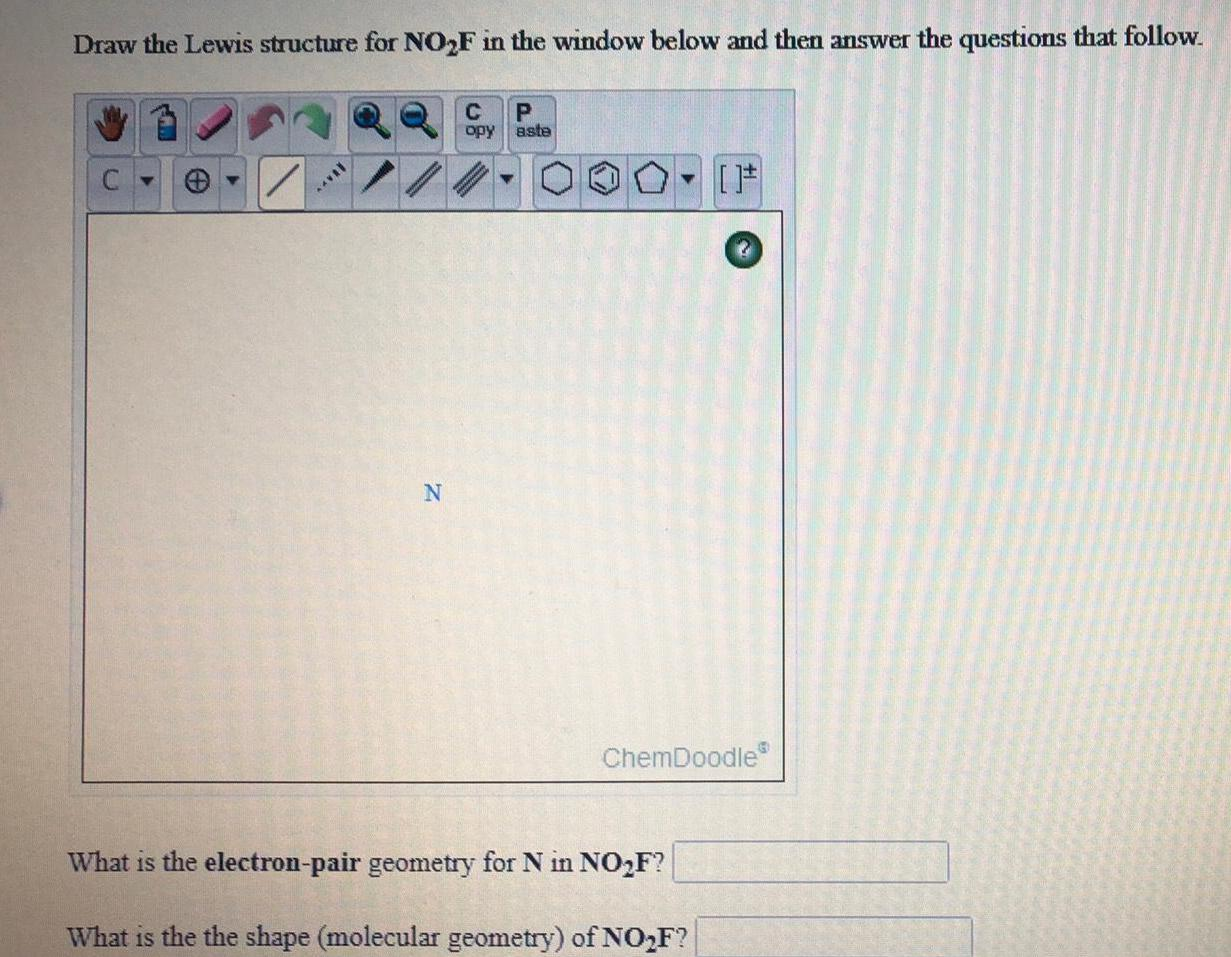Inorganic Chemistry
Hydrogen
Draw the Lewis structure for NO₂F in the window below and then answer the questions that follow. What is the electron-pair geometry for N in NO₂F? What is the the shape (molecular geometry) of NO₂F?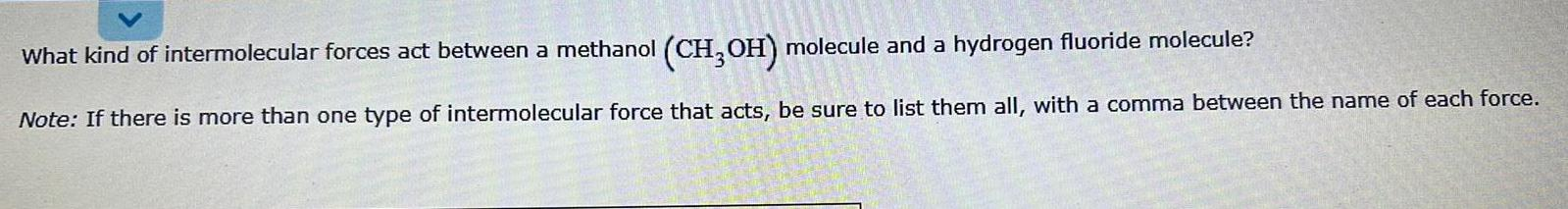Inorganic Chemistry
Hydrogen
What kind of intermolecular forces act between a methanol (CH3OH) molecule and a hydrogen fluoride molecule?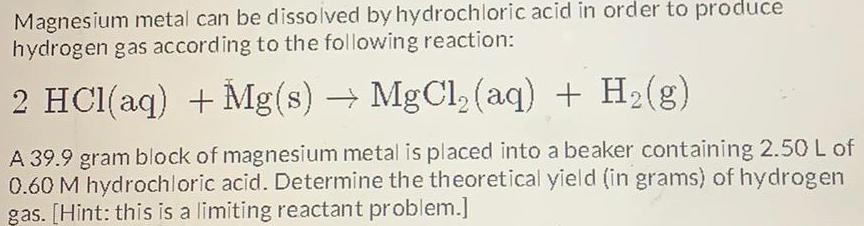Inorganic Chemistry
Hydrogen
Magnesium metal can be dissolved by hydrochloric acid in order to produce hydrogen gas according to the following reaction: 2 HCl(aq) + Mg(s) → MgCl₂ (aq) + H₂(g) A 39.9 gram block of magnesium metal is placed into a beaker containing 2.50 L of 0.60 M hydrochloric acid. Determine the theoretical yield (in grams) of hydrogen gas. [Hint: this is a limiting reactant problem.]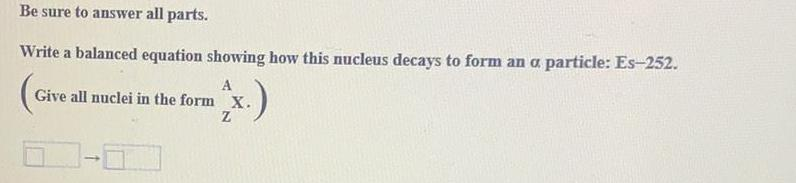Inorganic Chemistry
Hydrogen
Be sure to answer all parts. Write a balanced equation showing how this nucleus decays to form an a particle: Es-252. (Give all nuclei in the form AZX.)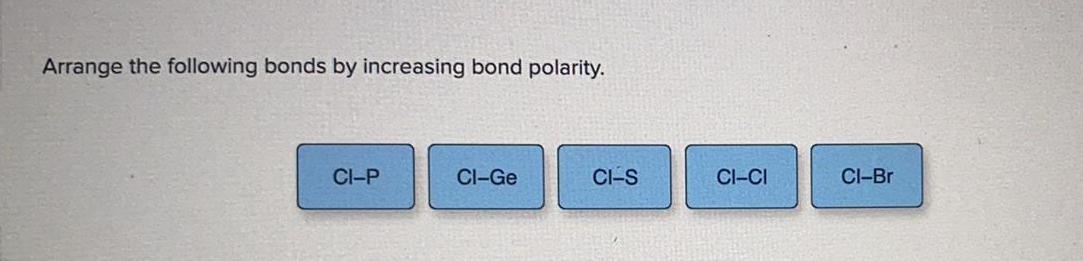Inorganic Chemistry
Hydrogen
Arrange the following bonds by increasing bond polarity. CI-P Cl-Ge CI-S CI-CI Cl-Br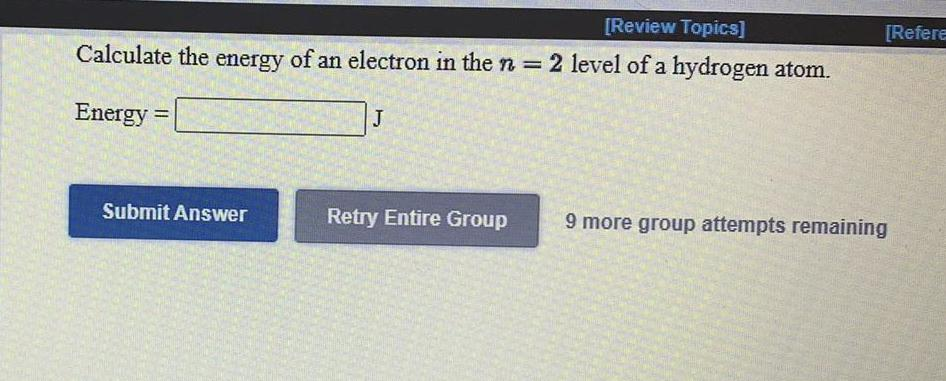Inorganic Chemistry
Hydrogen
Calculate the energy of an electron in the n = 2 level of a hydrogen atom.